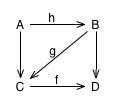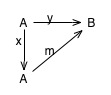# Category Diagrams

One of the most unusual things about category theory that I really love is category diagrams. In category theory, many things that would be expressed as equations or complex formulae in most mathematical formalisms can be presented as diagrams in category theory. If you are, like me, a very visual thinker, than category diagrams can present information in a remarkably clear form – and the categorical form of many statements of proofs can be much clearer than the alternatives because it can be presented in diagram form.

A category diagram is a directed graph, where the nodes are objects from a category, and the edges are morphisms. Category theorists say that a graph commutes if, for any two paths through arrows in the diagram from node A to node B, the composition of all edges from the first path is equal to the composition of all edges from the second path. (But it’s important to note that you do need to be careful here: merely because you can draw a diagram doesn’t mean that it necessarily commutes, just like being able to write an equation doesn’t mean that the equation is true! You do need to show that your diagram is correct and commutes.)

As usual, an example will make that clearer.This diagram is a way of expressing the associativy property of morphisms:$f circ (g circ h) = (f circ g) circ h$. The way that the diagram illustrates this is:$g circ h$ is the morphism from A to C. When we compose that with$f$, we wind up at D. Alternatively,$f circ g$ is the arrow from B to D; if we compose that with$h$, we wind up at D. The two paths:$f circ (A rightarrow C)$ and$(B rightarrow D) circ H$ are both paths from A to D, therefore if the diagram commutes, they must be equal. And the arrows on the diagram are all valid arrows, arranged in connections that do fit the rules of composition correctly, so the diagram does commute.

Let’s look at one more diagram, which we’ll use to define an interesting concept, the principal morphism between two objects. The principle morphism is a single arrow from A to B such that any composition of morphisms that goes from A to B will end up being equivalent to it.

In diagram form, a morphism m is principle if$forall x : A rightarrow A, forall y: A rightarrow B$, the following diagram commutes:In words, this says that$f$ is a principal morphism if for every endomorphic arrow$x$, and for every arrow$y$ from A to B,$f$ is is the result of composing$x$ and$y$. There’s also something interesting about this diagram that you should notice: A appears twice in the diagram! It’s the same object; we just draw it in two places to make the commutation pattern easier to see. A single object can appear in a diagram as many times as you want to to make the pattern of commutation easy to see. When you’re looking at a diagram, you need to be a bit careful to read the labels to make sure you know what it means.

One more definition by diagram:$(x, y)$ is a a retraction pair, and A is a retract of B (written$A < B$) if the following diagram commutes:That is,$x : A rightarrow B, y: B rightarrow A$ are a retraction pair if$y circ x = 1_A$.

## 2 thoughts on “Category Diagrams”

1.Pseudonym

You’ve made a mistake here, which is important, because it confused me when I was working through this a few years ago.

In the second diagram, A doesn’t appear twice just for clarity. Suppose that there were two nodes, A and B, with an arc from A to A labelled “x”, and two arcs from A to B labelled “y” and “m”. The property that the diagram commutes, would imply that xy = xm = y = m (amongst other identities, such as xxy=y).

In other words, A appears twice because if it only appeared once, the diagram would mean something different.

2.Mark Durst

Trivially — but annoying to those of us with terminal Proofreader’s Eye — maybe correct the twice-occurring misspelling”principle”?